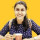1. Home >
2. Apps >
3. Groups >

# IIT JEE Mathematics Sample Paper - Algebra

Question asked by Ankita Katdare in #Coffee Room on May 30, 2010Ankita Katdare · May 30, 2010
Rank A1 - PRO
Algebra

1. [*]Let x be a real number with 0<x<p. Prove that, for all natural numbers n, the sum sinx + sin3x/3 + sin5x/5 + ... + sin(2n-1)x/(2n-1) is positive.
[*]Use combinatorial argument to prove the identity:
¥
å n-r+1Cd . r-1Cd-1 = nCr
d=1
[*]a, b are roots of x2+ax+b=0, g, d are roots of x2-ax+b-2=0. Given 1/a + 1/b + 1/d + 1/g =5/12 and abdg = 24, find the value of the coefficient ‘a’.
[*]x + y + z = 15 and xy + yz + zx = 72, prove that 3 £ x £ 7.
[*]Let l, a Î R, find all the set of all values of l for which the set of linear equations has a non-trivial solution.
lx + (sin a) y + (cos a) z = 0
x + (cos a) y + (sin a) z = 0
-x + (sin a) y - (cos a) z = 0
If l = 1, find all values of a.
[*]Prove that for each posive integer 'm' the smallest integer which exceeds (Ö3 + 1)2m is divisible by 2m+1.
[*]Prove that, for every natural k, the number (k3)! is divisible by (k!)k2+k+1.
[*]Prove that the inequality: n=1år { m=1år aman/(m+n)} ³ 0. ai is any real number.
[*]Prove the following inequality: k=1ån Ö[nCk] £ Ö[n(2n-1)]
[*]A sequence {Un, n ³ 0} is defined by U0=U1=1 and Un+2=Un+1+Un.Let A and B be natural numbers such that A19 divides B93 and B19 divides A93.Prove by mathematical induction, or otherwise, that the number (A4+B8)Un+1 is divisible by (AB)Un for n ³ 1.
[*]The real numbers a, b satisfy the equations: a3 + 3a2 + 5a - 17 = 0, b3 - 3b2 + 5b + 11 = 0. Find a+b.
[*]Given 6 numbers which satisfy the relations:
y2 + yz + z2 = a2
z2 + zx + x2 = b2
x2 + xy + y2 = c2
Determine the sum x+y+z in terms of a, b, c. Give geometrical interpretation if the numbers are all positive.
[*]Solve: 4x2/{1-Ö(1+2x2)}2 < 2x+9
[*]Find all real roots of: Ö(x2-p) + 2Ö(x2-1) = x
[*]The solutions a, b, g of the equation x3+ax+a=0, where 'a' is real and a¹0, satisfy a2/b + b2/g + g2/a = -8. Find a, b, g.
[*]If a, b, c are real numbers such that a2+b2+c2=1, prove the inequalities: -1/2 £ ab+bc+ca £ 1.
[*]Show that, if the real numbers a, b, c, A, B, C satisfy: aC-2bB+cA=0 and ac-b2>0 then AC-B2£0.
[*]When 0<x<1, find the sum of the infinite series: 1/(1-x)(1-x3) + x2/(1-x3)(1-x5) + x4/(1-x5)(1-x7) + ....
[*]Solve for x, y, z:
yz = a(y+z) + r
zx = a(z+x) + s
xy = a(x+y) + t
[*]Solve for x, n, r > 1
xCrn-1Crn-1Cr-1 x+1CrnCrnCr-1 x+2Crn+2Crn+2Cr-1 = 0
[*]Let p be a prime and m a positive integer. By mathematical induction on m, or otherwise, prove that whenever r is an integer such that p does not divide r, p divides mpCr.
[*]Let a and b be real numbers for which the equation x4 + ax3 + bx2 + ax + 1 = 0 has at least 1 real solution. For all such pairs (a,b), find the minimum value of a2+b2.
[*]Prove that:
2/(x2 - 1) + 4/(x2 - 4) + 6/(x2 - 9) + ... + 20/(x2 - 100) =
11/((x - 1)(x + 10)) + 11/((x - 2)(x + 9)) + ... + 11/((x - 10)(x + 1))
[*]Find all real p, q, a, b such that we have (2x-1)20 - (ax+b)20 = (x2+px+q)10 for all x.
Posted in: #Coffee RoomAnkita Katdare · May 30, 2010
Rank A1 - PRO
Some more Questions

1. Simplify the expression (a > 0, a ¹ 0) : (a-x/Ö 5)[2a2x-ax(2ax-1)] {1-(Ö5ax/2ax-1)-2}-1/2´ Ö[(ax+2)2-5]-(a2x+4)[a2x+4(1-ax)]-1/2+4ax[1+(ax+2)(a2x-4ax+4)-1/2]{ax+2+(a2x-4ax+4)1/2}-1 and determine for which values of x this expression is equal to 1.
2. Prve that log418 is an irrational number.
3. Determine all such integers a and b for which one of the roots of 3x3+ax2+bx+12=0 is equal to 1 + Ö3.
4. Solve in terms of complex numbers: z3 + (w7)*=0; z5.w11 = 1. (* indicates conjugate).
5. Prove that if a > 0, b > 0 then for any x and y the following inequality holds true: a.2x+b.3y+1 £ Ö(4x+9y+1)Ö(a2+b2+1)
6. Prove the inequality nn+1 > (n+1)n, n ³ 3, n Î N.
7. Prove that (b+c)2a2a2 b2(c+a)2b2 c2c2(a+b)2 = 2abc(a+b+c)3 (Without expanding)
8. Sum the series: 1 + 4x + 9x2 + ...
9. The eqns ax2 + bx + c=0 and x3=k have a common root. Prove that abc bcak cakbk = 0
10. If w is a root of x4=1 then Show that a + bw + cw2 + dw3 is a factor of abcd bcda cdab dabc Hence Show that the det is equal to -(a+b+c+d)(a-b+c-d){(a-c)2+(b-d)2}.
11. Find the coefficient of x4 in (1 + 2x + 3x2)5.
12. The sum of squares of 3 terms of a GP is S2. If their sum is aS, Prove that a2 Î (1/3,1) È (1,3).
13. Find the sum to n terms: 0!/5! + 1!/6! + 2!/7! + ...
14. If f(x)=ax/(ax + Öa) (a > 0), evaluate r=1å2n-1 f(r/2n).

## You must log-in or sign-up to reply to this post.

Click to Log-In or Sign-Up